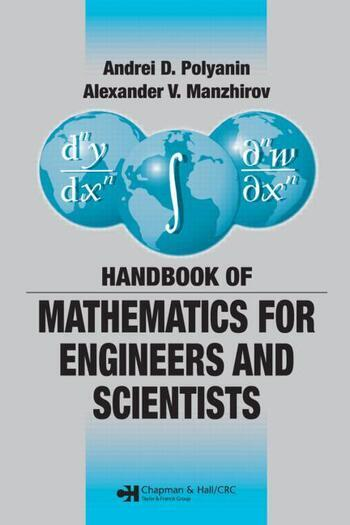Handbook of Mathematics for Engineers and Scientists

1st Edition

Andrei D. Polyanin, Alexander V. Manzhirov

Chapman and Hall/CRC
Published November 27, 2006
Reference - 1544 Pages - 226 B/W Illustrations
ISBN 9781584885023 - CAT# C5025

was \$145.00

USD\$116.00

SAVE ~\$29.00

FREE Standard Shipping!

Preview

Summary

The Handbook of Mathematics for Engineers and Scientists covers the main fields of mathematics and focuses on the methods used for obtaining solutions of various classes of mathematical equations that underlie the mathematical modeling of numerous phenomena and processes in science and technology. To accommodate different mathematical backgrounds, the preeminent authors outline the material in a simplified, schematic manner, avoiding special terminology wherever possible.

Organized in ascending order of complexity, the material is divided into two parts. The first part is a coherent survey of the most important definitions, formulas, equations, methods, and theorems. It covers arithmetic, elementary and analytic geometry, algebra, differential and integral calculus, special functions, calculus of variations, and probability theory. Numerous specific examples clarify the methods for solving problems and equations. The second part provides many in-depth mathematical tables, including those of exact solutions of various types of equations.

This concise, comprehensive compendium of mathematical definitions, formulas, and theorems provides the foundation for exploring scientific and technological phenomena.